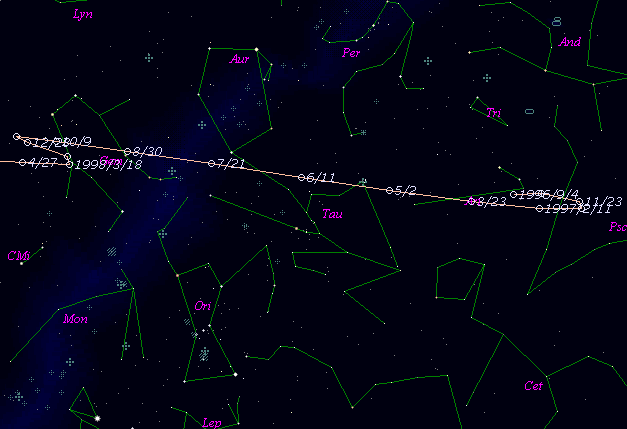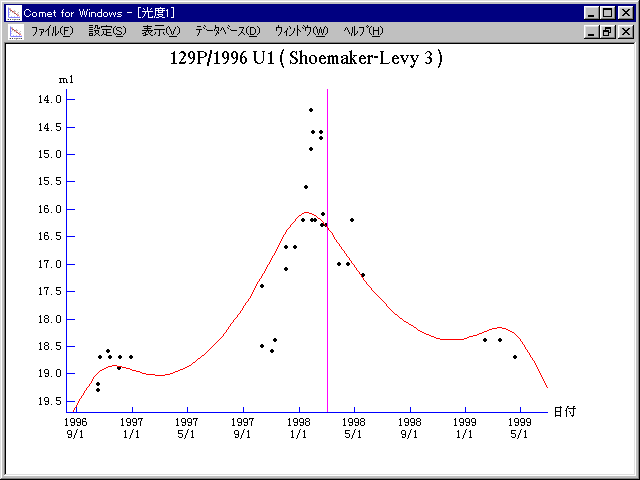# \$B%7%e!<%a!<%+!<(B-\$B%l%t%#Bh#3WB@1(B

129P/Shoemaker-Levy 3 (1998)###\$B%W%m%U%#!<%k(B

 \$BId9f(B 129P/1996 U1 \$B8!=PF|(B 1996\$BG/(B10\$B7n(B17\$BF|(B \$B8!=P8wEY(B 19.3\$BEy(B \$B8!=P G. Hahn, A. Maury, M. Lundstrom (Caussols)

###\$B50F;MWAG(B

```   The following improved orbital elements by Kenji Muraoka, are
from 225 observations 1991 Feb. 7 to 1999 Feb. 12, perturbations
by 9 Plantes, Moon and 5 minor planets were taken into account.
The mean residual is +/- 0.54 arc seconds.

Epoch  =  1998 Mar.  8.0  TT       JDT = 2450880.5
T  =  1998 Mar.  4.88686       +/- 0.00046 (m.e.) TT
Peri. =  181.38019                +/- 0.00013
Node  =  303.71158                +/- 0.00009   (2000.0)
Incl. =    5.00844                +/- 0.00002
q  =    2.8173477              +/- 0.0000013 AU
e  =    0.2479061              +/- 0.0000003
a  =    3.7460054              +/- 0.0000006 AU
n  =    0.13594132             +/- 0.00000003
P  =    7.250                  +/- 0.0000018  years
```

###\$B@1?^(B###\$B8wEYJQ2=(B

```        m1 = 5.7 + 5 log\$B&\$(B + 20.0 log r
```##### \$B50F;MWAG\$OB<2,7r<#;a\$N7W;;\$K\$h\$k\$b\$N\$G\$9!#(B \$B@1?^\$O(B StellaNavigator Ver.2.0 for Windows (\$B%"%9%H%m%"!<%D(B \$BJTCx(B / \$B%"%9%-!<=PHG6I4)(B) \$B\$G:n@.\$7\$?\$b\$N\$G\$9!#(B \$B8wEY%0%i%U\$O(BComet for Windows\$B\$G:n@.\$7\$?\$b\$N\$G\$9!#(B